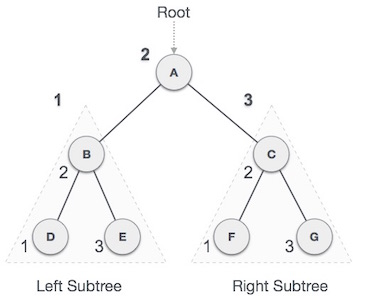# Tree Traversal

Traversal is the process of visiting all the nodes of a tree and printing their values. We always start from the root (head) node since all nodes are connected via edges (links). That is, we cannot reach a node in a tree at random. There are three methods for traversing a tree:

• In-order Traversal
• Pre-order Traversal
• Post-order Traversal

In-order Traversal

This traversal technique visits the left subtree first, then the root, and finally the right subtree. It is important to note that every node might represent a subtree.

If a binary tree is traversed in ascending order, the result will be sorted key values.We start from A, and following in-order traversal, we move to its left subtree B. B is also traversed in-order. The process goes on until all the nodes are visited. The output of inorder traversal of this tree will be −D → B → E → A → F → C → G

Pre-order Traversal

In this traversal method, the root node is visited first, then the left subtree and finally the right subtree.

We start from A, and following pre-order traversal, we first visit A itself and then move to its left subtree BB is also traversed pre-order. The process goes on until all the nodes are visited. The output of the pre-order traversal of this tree will be −

A → B → D → E → C → F → G

Post-order Traversal

The root node is inspected last in this traversal mode, thus the name. We go through the left subtree first, then the right subtree, and lastly the root node.

We start from A, and following Post-order traversal, we first visit the left subtree BB is also traversed post-order. The process goes on until all the nodes are visited. The output of the post-order traversal of this tree will be −

D → E → B → F → G → C → A

0